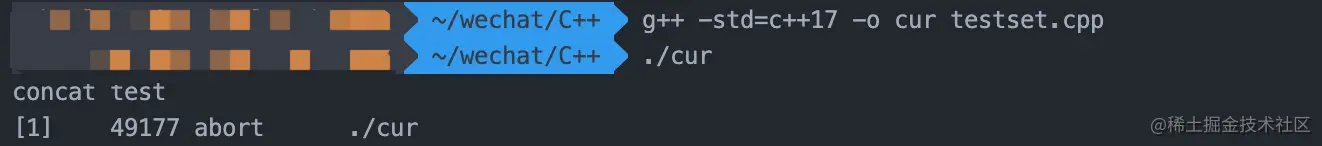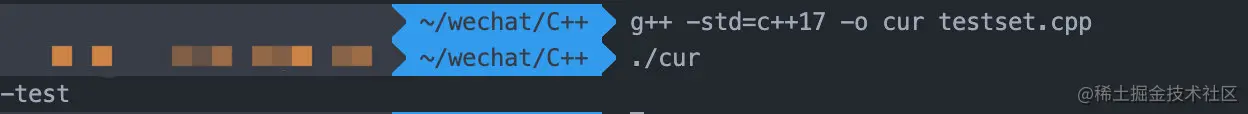# EasyC++11，cstring.h函数大全

## cstring.h常用函数

cstring.h库即C语言中的string.h库，它是C语言中为字符串提供的标准库。C++对此进行了兼容，所以我们在C++当中一样可以使用。

### strlen

``````char name = "hello world";
cout << strlen(name) << endl;

### strcat

`strcat`函数可以将两个字符串进行拼接，它的函数签名为：

``````char *strcat(char *dest, const char *src)

``````char name = "hello world";
char level = "concat test";

char *ret = strcat(name, level);
cout << ret << endl;	// 方式1
cout << name << endl;	// 方式2

### strncat

`strcat`函数的变种，函数额外多接收一个参数控制拷贝`src`字符串的最大长度。

``````char *strncat(char *dest, const char *src, size_t n)

``````char name = "hello world";
char level = "concat test";

char *ret = strncat(name, level, 4);
cout << ret << endl;
cout << name << endl;

### strcpy

``````char *strcpy(char *dest, const char *src)

1. 如果`dest`字符串长度不够长，在编译时不会报错，但运行时可能导致问题。
``````char name = "";
char level = "concat test";

strcpy(name, level);
cout << name << endl;1. 如果`dest`中原本就有内容，会被覆盖。
``````char name = "abc";
char level = "concat test";

strcpy(name, level);
cout << name << endl;

### strncpy

`strcpy`加入长度限制的版本，可额外多传入一个参数n表示最多赋值n个字符。当`src`长度小于n时，剩余部分将会使用空字节填充。

``````char *strncpy(char *dest, const char *src, size_t n)

``````char name = "abc";
char level = "concat test";

strncpy(name, level, 4);
cout << name << endl;

### memset

``````void *memset(void *str, int c, size_t n)

`int c`表示要被设置的字符，`size_t n`表示设置的字符数量。

``````char name = "abc";
char level = "concat test";

memset(name, 'c', 10);
cout << name << endl;

``````int a;
memset(a, 1, sizeof a); // memset(a, 1, 400); 因为一个int占据4个字节

``````// 1. 批量设置成0
memset(a, 0, sizeof a);
// 2. 批量设置成-1
memset(a, -1, sizeof a);
// 3. 批量设置成一个接近最大整数上限的值
memset(a, 0x7f, sizeof a);
memset(a, 0x3f, sizeof a);

### memcpy

`strcpy`类似，从`str2`中复制N个字符到`str1`中。

``````void *memcpy(void *str1, const void *str2, size_t n)

``````char name = "abc";
char level = "concat test";

memcpy(name, level, 10);

### strcmp

``````int strcmp(const char *str1, const char *str2)

``````char name = "abc";
char level = "abcd";

cout << strcmp(name, level) << endl;

### strncmp

`strcmp`长度限制版，可以额外接受一个数字n，表示最多比较长度为n的字符。

``````int strncmp(const char *str1, const char *str2, size_t n)

### strstr

``````char *strstr(const char *haystack, const char *needle)

`haystack`中搜索`needle`第一次出现的位置，返回指向该位置的指针。

``````char name = "search-test";
char level = "-";

char *ret = strstr(name, level);
cout << ret << endl;